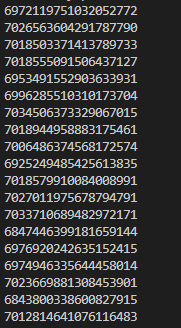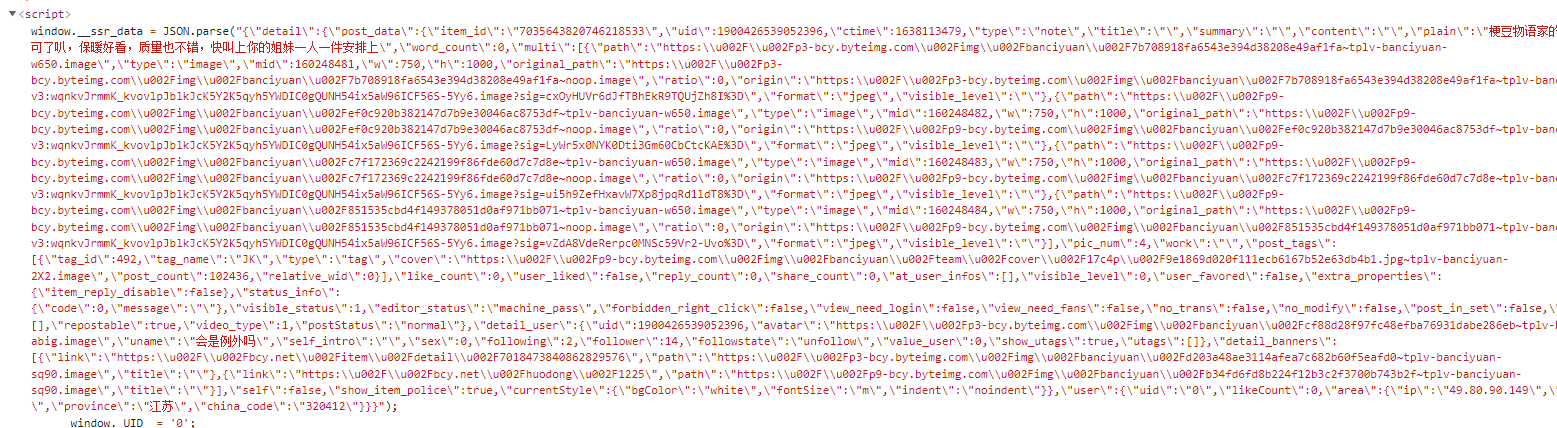### 获取列表数据`123456789101112131415161718` ``````import requests import time header = { 'user-agent': 'Mozilla/5.0 (Windows NT 10.0; Win64; x64) AppleWebKit/537.36 (KHTML, like Gecko) Chrome/96.0.4664.45 Safari/537.36' } def get_list(): try: url = 'https://bcy.net/apiv3/common/circleFeed?circle_id=492&since='+str(int(time.time()))+'.000000&sort_type=2&grid_type=10' response = requests.get(url,headers= header) response.raise_for_status() #转码 response.encoding = 'utf-8' print(response.text) except: print("Failed!") ``````

 `123456` ``````def parse_list(data): item_ids = [] json_data = json.loads(data) for item in json_data['data']['items']: item_ids.append(item['item_detail']['item_id']) return item_ids ``````### 获取 jk 图`1234567891011121314151617181920212223242526` ``````import re def get_item(item_ids): intercepts = [] for id in item_ids: url = 'https://bcy.net/item/detail/'+ str(id) + '?_source_page=hashtag' response = requests.get(url, headers = header) response.encoding = 'utf-8' text = response.text intercept = text[text.index('JSON.parse("') + len('JSON.parse("'): text.index('");')].replace(r'\"',r'"') intercepts.append(intercept) return intercepts def download(intercepts): for i in intercepts: pattern = re.compile('"multi":\[{"path":"(.*?)","type"') pattern_item_id = re.compile('"post_data":{"item_id":"(.*?)","uid"') b = pattern.findall(i) item_id = pattern_item_id.findall(i) index = 0 for url in b: index = index + 1 content = re.sub(r'(\u[a-zA-Z0-9]{4})',lambda x:x.group(1).encode("utf-8").decode("unicode-escape"),url) response = requests.get(content.replace('\','')) with open('D:\bcy\' + str(item_id) + str(index) + '.png', 'wb') as f: f.write(response.content) ``````

### 总结